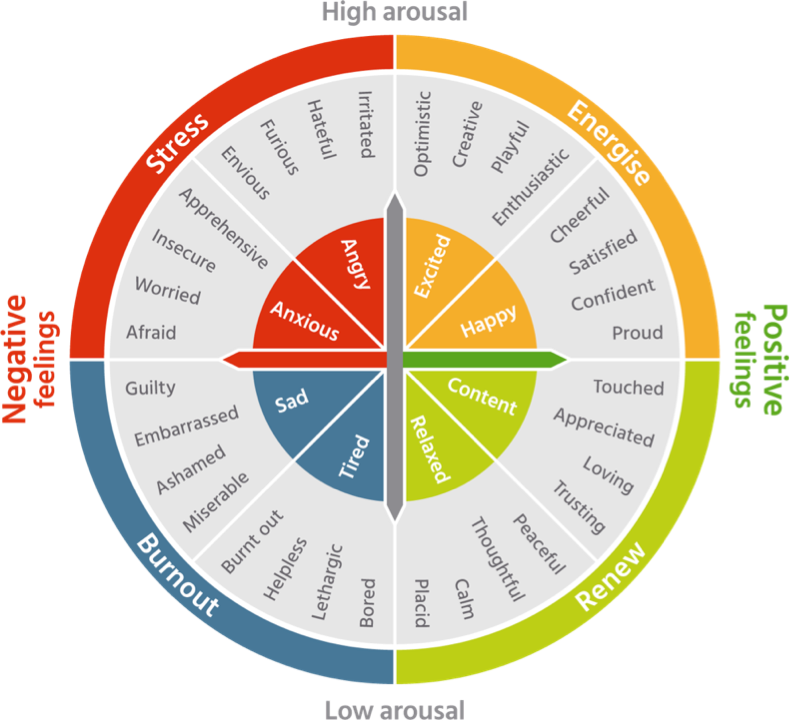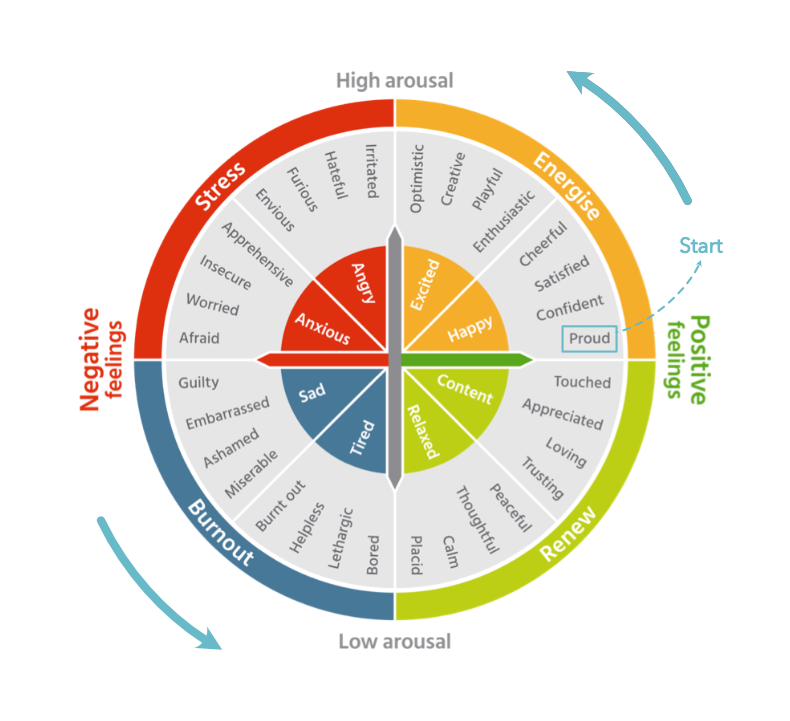Moody Me
Share
ExploreGetting started

#How it works

Concept and some explanations
The idea 💡
Here’s once again the “Wheel of Emotions” on which the mood tracker ( ) is based 😊.
You won’t see the wheel, but I kept the colours, the emotions, the octants and quadrants.
Everything you see here has been converted into tables and lookups 😊.How ? 🤔
If you take a look at the wheel, it can be divided into 2 distinctive parts :
The Emotions:
Their names, colours, grouping ...
The Wheel:
It’s nothing other than a . It can then be used to determine the precise position of each Emotion (i.e.: give each one of them unique ).
Unit Circle ? Cartesian coordinates ? 🤨
Yes, I know 😅!
From Wikipedia:
In , a unit circle is a of unit —that is, a radius of 1. Frequently, especially in , the unit circle is the circle of radius 1 centered at the origin (0, 0) in the in the .
In summary : It’s a circle with a radius of 1 and a point of origin at (0,0) in the Cartesian Coordinate system.
Illustration of a Cartesian coordinate plane.
Four points are marked and labeled with their coordinates:
(2, 3) in green, (−3, 1) in red, (−1.5, −2.5) in blue, and the origin (0, 0) in purple. -
Using trigonometric functions on the unit circle (also from ) :
The cosine and sine of angle θ may be defined on the unit circle as follows:

If (𝑥, 𝑦) is a point on the unit circle, and if the ray from the origin (0, 0) to (𝑥, 𝑦) makes an θ from the positive x-axis, (where counterclockwise turning is positive), then

cos θ = 𝑥 and sin θ = 𝑦
Illustration of a unit circle.
The variable t is an measure. -
Applying all this to the WheelWe can tell that :
The 𝑥 is the Positive feelings / Negative feelings axis (
@Feeling
)
The 𝑦 is the High arousal / Low arousal axis (
@Energy
)
There are 32 segments on this wheel corresponding to 32 Emotions (in the
@Spectrum 1 - 1⁄𝑥
which are then grouped by octants (
@Spectrum 2 - ⅛
@Spectrum 3 - ¼
))
As a full rotation of a circle is always 360°, we can easily determine the max angle θ for each segment (corresponding to the point where a segment ends and the new one starts).
As we can determine the angle θ, once converted in radians and using the , we can also determine the cosine and sine of each angle, giving us our needed Cartesian Coordinates (𝑥,𝑦).

Note: Because we’ve entered the trigonometry side of maths, the circle starts at Proud on the wheel and we are turning counterclockwise. So it goes from Proud to Confident, to Satisfied, etc... 😊All of this is done in the base table you’ll find in 😊.
Now what ? 🤔
Well, as we’re already in a unit circle, using cosine and sine, we can also create user-friendly readable scales (/10) for our axes 𝑥 and 𝑦 😊.
This allows us, when using the tracker, to retrieve any given segment (circular sector) on the wheel.
This is possible thanks to the fact that trigonometric functions, such as sine and cosine, react in a very specific way in each quadrant of a unit circle 😊, as shown in this chart used to convert degrees to radians and vice-versa ().
As you can see
0
Cosine ⟨𝑥⟩
Sine ⟨𝑦⟩
1
+
Decreasing
+
Increasing
2
-
Decreasing
+
Decreasing
3
-
Increasing
-
Decreasing
4
+
Increasing
-
Increasing
There are no rows in this table

By knowing this, we can, regardless of the Emotions, mathematically determine quadrants in our Wheel and sort out, accordingly, our sines and cosines.
We can also know precisely how many negative and positive values (as we’re in a Cartesian Coordinate System) are needed in each scale ( and ).
Both scales are then linked to a particular segment/Circular Sector and to each other.
Emotions are all stored in one table. It is as simple as that 😊.
But, if the table is the headquarters for everything related to the Wheel, Emotions have their counterpart in .
It is a bridge, a dashboard, echoing on the Emotion’s side which occurs in the Wheel, actively linking each segment/Circular Sectors to each emotions.
A few other things you should know 😊
Rows in those tables will be “Activate/Deactivate” if or when you activate/deactivate any emotions in the
@Spectrum 1 - 1⁄𝑥
(which is the most important one)
Activating/Deactivating emotions is there so you shouldn’t need to delete any emotions (for the sake of data integrity).
But, it might require patience as it relies on the Trigonometry pack which can be a bit slow when it comes to calculating sines and cosines.
You can re-order Emotions from to your liking. Their coordinates will be updated to their new position.
This means that if you wish to re-assign, mix and match some emotions, you would need to head to and change it there.
You can also rename Emotions, change their emoji... The colours are attributed through conditional formatting in the base table 😊
And you can add Emotions too 😊!
But because of the “dynamic nature” of some tables, adding emotions might require to also add rows in , , and .
Fortunately, there’s also a table in which can do that for you 😊.

Share